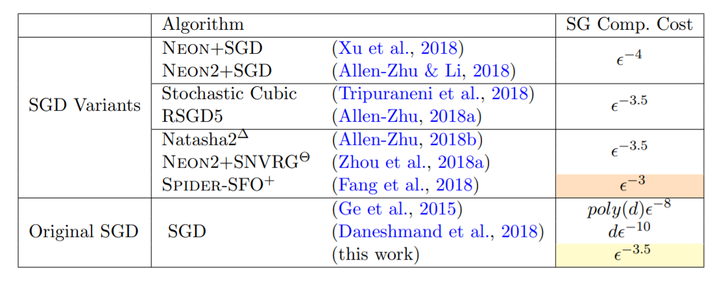# Sharp Analysis for Nonconvex SGD Escaping from Saddle Points### Abstract

In this paper, we prove that the simplest Stochastic Gradient Descent (SGD) algorithm is able to efficiently escape from saddle points and find an (eps, O(eps^0.5))-approximate second-order stationary point in O˜(eps^-3.5) stochastic gradient computations for generic nonconvex optimization problems, under both gradient-Lipschitz and Hessian-Lipschitz assumptions. This unexpected result subverts the classical belief that SGD requires at least O(eps−4) stochastic gradient computations for obtaining an (eps, O(eps0.5))-approximate second-order stationary point. Such SGD rate matches, up to a polylogarithmic factor of problem-dependent parameters, the rate of most accelerated nonconvex stochastic optimization algorithms that adopt additional techniques, such as Nesterov’s momentum acceleration, negative curvature search, as well as quadratic and cubic regularization tricks. Our novel analysis gives new insights into nonconvex SGD and can be potentially generalized to a broad class of stochastic optimization algorithms.

Type
Publication
Association for Computational Learning